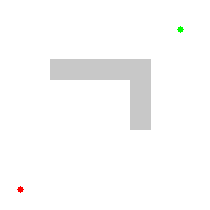# Introduction to A*

suggest change

A* (A star) is a search algorithm that is used for finding path from one node to another. So it can be compared with Breadth First Search, or Dijkstra’s algorithm, or Depth First Search, or Best First Search. A* algorithm is widely used in graph search for being better in efficiency and accuracy, where graph pre-processing is not an option.

A* is a an specialization of Best First Search , in which the function of evaluation f is define in a particular way.

f(n) = g(n) + h(n) is the minimum cost since the initial node to the objectives conditioned to go thought node n.

g(n) is the minimum cost from the initial node to n.

h(n) is the minimum cost from n to the closest objective to n

A* is an informed search algorithm and it always guarantees to find the smallest path (path with minimum cost) in the least possible time (if uses admissible heuristic). So it is both complete and optimal. The following animation demonstrates A* search-